﻿ How to Calculate the Emitter Current, Ie, of a Transistor# How to Calculate the Emitter Current, Ie, of a TransistorThe emitter current, Ie, of a transistor is the amplified output current of a bipolar junction transistor.

There are several ways to find the emitter current, Ie, of a transistor. And it all depends on what information is already known about the transistor:

### 1st Way To Calculate Emitter Current Ie

DC Analysis

Doing DC analysis of the transistor circuit is the most common way of finding out the value of Ie in the circuit.The equation to solve for Ie is: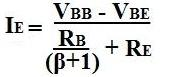So we must solve for Vbb and RB in order to solve for Ie.

The value of Vbb is computed by: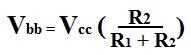Next we compute the value of RB:Now we can calculate the value of the emitter current, Ie, in the circuit:Example

Using the example of the values for the resistors and the voltage, Vcc, above, we're going to solve for Ieq.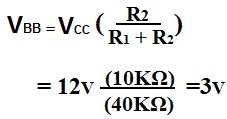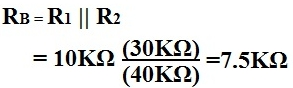### 2nd Way to Calculate Emitter Current Ie

Using Known Values
If Ib (the base current) and β are known, Ie can be solved for by using the formula:Example

If Ib=30µA and β=99, then the answer to the equation is:### 3rd Way to Calculate Emitter Current Ie

Using Known Values
If Ic and β are known, then Ie can be calculated by the formula:Example

If Ic= 2.97ma and β=99, then Ie is calculated out to be:### 4th Way to Calculate Emitter Current Ie

Using Known Values
If Ib and Ic are known, Ie can be calculated using the formula:Example
If Ic=3.95mA and Ib=50µA, then Ie calculates out to be: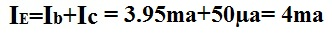Related Resources